# Signal Modulation

## Discrete Semiconductor Devices and Circuits

• #### Question 1

A modern method of electrical power control involves inserting a fast-operating switch in-line with an electrical load, to switch power on and off to it very rapidly over time. Usually, a solid-state device such as a transistor is used: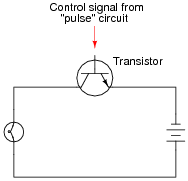This circuit has been greatly simplified from that of a real, pulse-control power circuit. Just the transistor is shown (and not the “pulse” circuit which is needed to command it to turn on and off) for simplicity. All you need to be aware of is the fact that the transistor operates like a simple, single-pole single-throw (SPST) switch, except that it is controlled by an electrical current rather than by a mechanical force, and that it is able to switch on and off millions of times per second without wear or fatigue.

If the transistor is pulsed on and off fast enough, power to the light bulb may be varied as smoothly as if controlled by a variable resistor. However, there is very little energy wasted when using a fast-switching transistor to control electrical power, unlike when a variable resistance is used for the same task. This mode of electrical power control is commonly referred to as Pulse-Width Modulation, or PWM.

Explain why PWM power control is much more efficient than controlling load power by using a series resistance.

• #### Question 2

Explain the difference between AM (Amplitude Modulation) and FM (Frequency Modulation).

• #### Question 3

A very important concept in electronics is modulation. Explain what “modulation” means, and give one or two examples of it.

• #### Question 4

A primitive form of communication long ago was the use of smoke signals: interrupting the rising stream of smoke from a fire by waving a blanket over it so that specific sequences of smoke “puffs” could be seen some distance away. Explain how this is an example of modulation, albeit in a non-electronic form.

• #### Question 5

One of the simplest electronic methods of modulation is amplitude modulation, or AM. Explain how a high-frequency carrier signal would be modulated by a lower-frequency signal such as in the case of the two signals shown here in the time domain: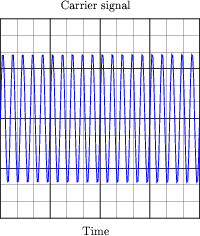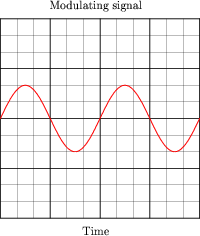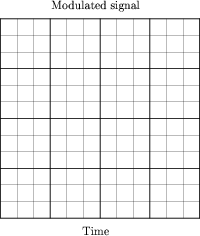• #### Question 6

A circuit often used to amplitude-modulate a carrier signal is a multiplier: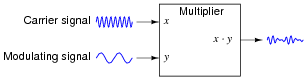Explain how the instantaneous multiplication of two sine waves results in amplitude modulation. If possible, graph this on a graphing calculator or other computer plotting device.

• #### Question 7

A common modulation technique employed in radio broadcasting is frequency modulation, or FM. Explain how a high-frequency carrier signal would be modulated by a lower-frequency signal such as in the case of the two signals shown here in the time domain: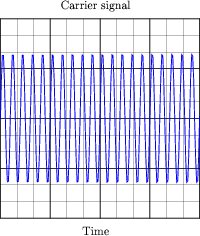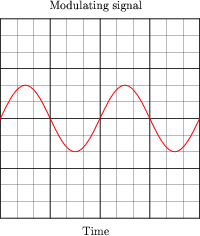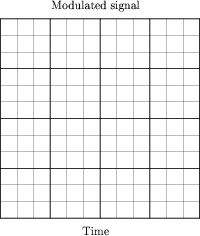• #### Question 8

At the heart of an FM transmitter is a circuit called a voltage-controlled oscillator, or VCO. Explain what the purpose of a VCO is, and how this directly relates to frequency modulation.

• #### Question 9

This is a schematic for a very simple VCO: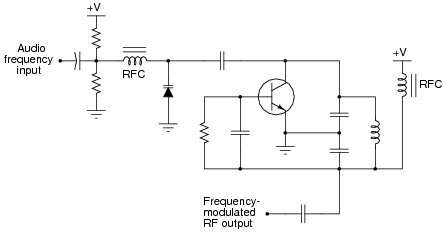The oscillator is of the “Colpitts” design. The key to understanding this circuit’s operation is knowing how the varactor diode responds to different amounts of DC bias voltage. Explain how this circuit works, especially how the diode exerts control over the oscillation frequency. Why does the output frequency vary as the control voltage varies? Does the output frequency increase or decrease as the control voltage input receives a more positive voltage?

Note: “RFC” is an acronym standing for Radio-Frequency Choke, an iron-core inductor whose purpose it is to block radio frequency current from passing through.

• #### Question 10

This is a schematic for a simple VCO:The oscillator is of the RC “phase shift” design. Explain how this circuit works. Why does the output frequency vary as the control voltage varies? Does the output frequency increase or decrease as the control voltage input receives a more positive voltage?

Hint: the JFETs in this circuit are not functioning as amplifiers!

• #### Question 11

FM tends to be a far more noise-resistant means of signal modulation than AM. For instance, the “crackling” form of radio interference caused by natural lightning or the “buzzing” noise produced by high-voltage power lines are both easy to hear on an AM radio, but absent on an FM radio. Explain why.

• #### Question 12

When transmitting audio information (such as music and speech) in the form of radio waves, why bother modulating a high-frequency carrier signal? Why not just connect a powerful audio amplifier straight to an antenna and broadcast the audio frequencies directly?

• #### Question 13

Predict how the output frequency of this voltage-controlled oscillator (VCO) circuit will be affected as a result of the following faults. Consider each fault independently (i.e. one at a time, no multiple faults):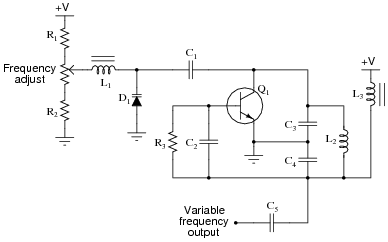Capacitor C1 fails open:
Inductor L1 fails open:
Resistor R1 fails open:
Resistor R2 fails open:
Inductor L2 fails partially shorted:

For each of these conditions, explain why the resulting effects will occur. Note: the voltage-dependent capacitance of a varactor diode is given by the following equation:

 $$C_j = \frac{C_o}{\sqrt{2V+1}}$$

Where,

CJ = Junction capacitance

Co = Junction capacitance with no applied voltage

V = Applied reverse junction voltage

• #### Question 14

Determine the duty cycle of this square wave signal: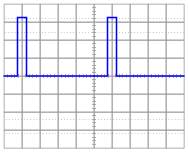• #### Question 15

Determine the duty cycle of this square wave signal: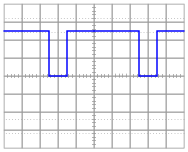• #### Question 16

A resistive DC load receives pulse-width modulated (PWM) power from a controller circuit, and an oscilloscope shows the load voltage waveform as such: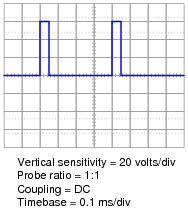Calculate the duty cycle of this waveform, and also the average power dissipated by the load assuming a load resistance of 1.8 Ω.

• #### Question 17

A resistive DC load receives pulse-width modulated (PWM) power from a controller circuit, and an oscilloscope shows the load voltage waveform as such: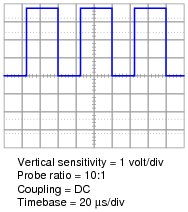Calculate the duty cycle of this waveform, and also the average power dissipated by the load assuming a load resistance of 10.3 Ω.

• #### Question 18

The oscillator circuit in this diagram generates a square wave with an adjustable duty cycle: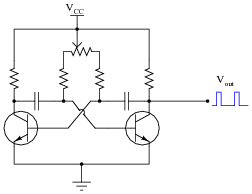A student desires to use this circuit as the basis for a pulse-width modulation (PWM) power controller, to vary the amount of power delivered to a DC load. Since the oscillator circuit is built to produce weak signals and not deliver power directly to a load, the student adds a power MOSFET to switch heavy load currents: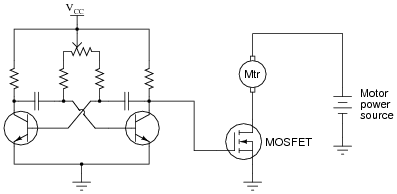Correlate the duty cycle of the oscillator’s output signal with motor power. In other words, describe how increases and decreases in signal duty cycle affect the amount of power delivered to the electric motor.

• #### Question 19

Explain why it is important for the final power transistor(s) in a PWM power control circuit to operate at full cutoff and full saturation, and not in the linear (active) mode in between those two extremes. What might happen if the power transistor(s) were to be less than cut-off or less than saturated when carrying load current?

• #### Question 20

If a pulse-width modulated (PWM) signal is sent to a passive integrator circuit from a circuit capable of both sourcing and sinking current (as is the case with the dual-MOSFET output stage), the output will be a DC voltage (with some ripple):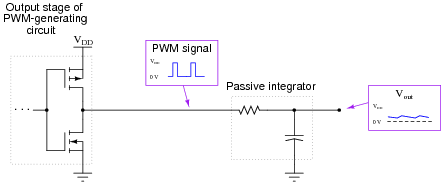Determine the relationship between the PWM signal’s duty cycle and the DC voltage output by the integrator. What does this suggest about PWM as a means of communicating information, such as analog data from a measuring device?

• #### Question 21

Plot what the frequency spectrum would look like for a pure (undistorted) 1 MHz sine wave:• #### Question 22

Determine the frequency spectrum for a high-frequency, sine wave “carrier” signal that is amplitude-modulated (AM) by an audio-frequency sine wave signal, as the following block diagram shows: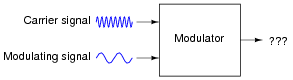The spectra for these respective waveforms are shown individually: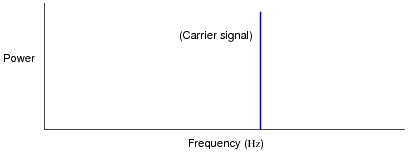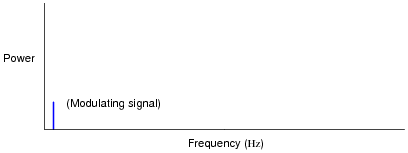Plot the modulated signal spectrum here: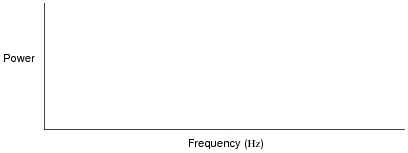• #### Question 23

Determine the frequency spectrum for a high-frequency, sine wave “carrier” signal that is amplitude-modulated (AM) by an audio-frequency signal with a spectrum of its own. The spectra for these respective waveforms are shown individually: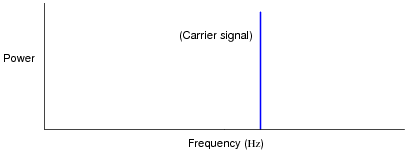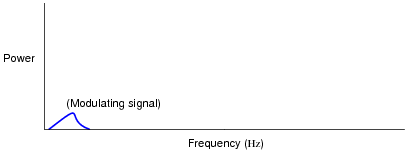Plot the modulated signal spectrum here: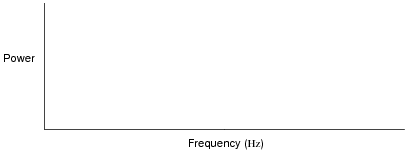• #### Question 24

An important measurement of pulse waveforms is duty cycle. Give a precise. mathematical definition for this term.

Also, write an equation solving for pulse width given duty cycle (D) and frequency (f).

• #### Question 25

Determine the duty cycle of this square wave signal: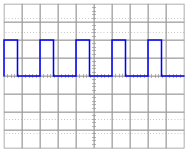• #### Question 26

Determine the duty cycle of this square wave signal: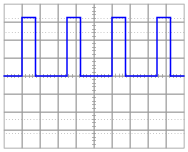• #### Question 27

How would a permanent-magnet DC motor respond if the switch in this circuit were repeatedly closed and opened at a very high frequency?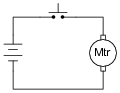Would it rotate at full speed, just the same as if the switch were closed all the time? Would it rotate at all? Explain your answer.

• #### Question 28

A resistive DC load receives pulse-width modulated (PWM) power from a controller circuit, and an oscilloscope shows the load voltage waveform as such: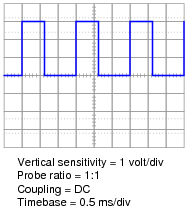Calculate the duty cycle of this waveform, and also the average power dissipated by the load assuming a load resistance of 2.5 Ω.

• #### Question 29

A resistive DC load receives pulse-width modulated (PWM) power from a controller circuit, and an oscilloscope shows the load voltage waveform as such: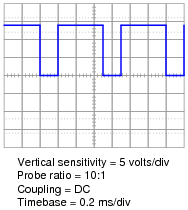Calculate the duty cycle of this waveform, and also the average power dissipated by the load assuming a load resistance of 40.7 Ω.

• #### Question 30

How is pulse-width modulation power control similar to the form of control exerted by TRIACs and SCRs in AC power circuits? How does it differ?